# Neural Networks Demystified

One of the most powerful and widely used artificial-intelligence approaches is called neural networks. But, What exactly are they? and How they work? Let me explain it in plain English.

# What is a Neural Network?

A neural network is a collection of connected nodes called neurons.

# What is a Neuron?

A neuron is a node that has one or more inputs and a single output, as shown in Figure 1. Three important actions take place inside of a neuron:

• A weight is associated with each input to amplify or de-amplify it.
• To calculate the output, all weighted inputs are summed.
• The result of the sum is used as an input for an activation function which determines the final output.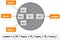Figure 1. A neuron is like an equation –it associates a weight to each of its inputs and calculates an output adding the weighted inputs and applying an activation function.

# What is an Activation Function?

An activation function is a mathematical equation that outputs a small value for small inputs, and a larger value if its inputs exceed a threshold. An example of activation fuction, which is commonly used, is the sigmoid function shown in Figure 2.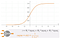Figure 2. The sigmoid function is commonly used as the neuron’s activation function usinng as input the sum of all weighted neuron’s inputs.

The idea is quite simple: input values that are close to zero will cause a large change in the output, while input values too big or too small will cause a very small difference.

# How Are Neurons Connected?

The output of one neuron can be used as an input to other neurons. Typically, neurons are aggregated into layers. Layer is a general term that applies to a collection of nodes operating together at a specific depth within the neural network. Outputs travel from the first layer, to the last layer. As shown in Figure 3, there is typically an input Layer, one or more middle layers (called hidden layers), and an output Layer.

• The input layer contains only data. There are not working neurons there.
• The hidden layer(s) is/are where the learning occurs — later we will review how.
• The output layer contains neurons that calculate the final output.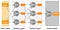Figure 3. A neural network with two inputs in an input layer, two hidden layers, and one neuron in the output layer.

The number of input and output neurons is dependent upon the problem at hand. The number of hidden neurons is often the sum of the number of input and output neurons, but it is not a rule.

# How Do They Work?

Neural networks help us to classify information. They are trained (learn) by processing examples, each of which contains a known input and output. The result of the training process is to calculate the values for the weights associated with each input in each neuron. Once we train the neural network, i.e., we calculate the values for all the weights, we can use the neural network for mapping new unseen inputs to an output.

# Example

The Hello-World example for neural networks is usually the implementation of a neural network to recognize the XOR operator. The neural network for this has

• two inputs,
• one output,
• and, we will use one hidden layer with three neurons — as it recommended, the sum of input and output neurons.

Our neural network is shown in Figure 4, as well as the input data that we will use to train the network and the known outputs.

## Step 1. Initialize Weights and Bias

The first step with a neural network is to initialize weights. What options do we have?

• Initialize with zeros only — it would be a poor strategy 😳. Remember, weight is going to be multiplied by the inputs, so with wights equal to zero the inputs no longer play a role, and the neural network cannot learn properly.
• Initialize weights randomly – it is a bit naïve, but it works nicely very often, except in a few cases. Let’s use this approach for our example.

Thus, we are going to initialize the nine weight values in our neural network with random values.

## Step 2. Forward Propagation

It is a fancy name for providing the network with an input and observing the output. We start at the input layer and calculate the outputs for the hidden layer. The results are passed forward to the next layer. Then, we calculate the output in the output layer using the outputs from the hidden layer as inputs. Figure 5 shows the maths. Just linear algebra. That’s it.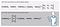Figure 5. Calculate the outputs for each neuron, starting at the input layer and move forward; use the outputs from the neurons in one layer as the inputs for the neurons in the next one.

## Step 3. Calculate the Error

The error is calculated as the difference between the known output and the calculated output (output ₃ in our example). Error values are commonly square to remove negative signs and give more weight to larger differences. A division by 2 does not affect the calculation and will be useful later for making the derivative simpler.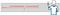Figure 6. Error is calculated as the difference between the known output and the calculated output.

If the neural network has more than one node in the output layer, the error is calculated as the addition of all the partial errors.

## Step 4. Backward Propagation

Since we are using random values for the weights, it is highly probable that our output will have a high error. We need to reduce the error. The only way to reduce the error is to change calculated value. And, the only way to change the calculated value is by modifying the values of the weights. A proper adjustment of weigths ensures that the subsequent output will be closer to the expected output. This process is repeated until we are satisfied that the network can produce results significantly close enough to the known output.

How to modify the value of the weights so that the error is reduced?

Short answer: use the gradient descent algorithm. It was first sugested in 1847. It applies multivariable calculus, specifically partial derivatives. The derivative of the error function with respect to each weight is used to adjust the weights values. The derivative of the error function can be multiplied by a selected number (called learning rate) to make sure that the new updated weight is minimizing the error function. The learning rate is a small positive value, often in the range between 0.0 and 1.0.

To calculate the partial derivatives with respect to the weights, the derivative of the error function and the derivative of the sigmod function are needed. Figure 7 shows the general equation for the weights update and one example solving the equation for the weight W₆ — the weight of the first input for the neuron in the output layer.

The calculus chain rule principle is applied to compute the derivative of the composite function. Be aware that calculations are similar but not the same for neurons in the output layer and neurons in the hidden layer.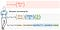Figure 7. Equations to: update the weight values (in red), error and derivative of the error (in gray), and calculated output (sigmoid function) derivative.

• we calculate outputs for all neurons using the math in Figure 5 (forward propagation) and the difference between the calculated output and the known output (error).
• If the difference is greater than what we expected, we calculate new weight values (backward propagation).

These two activities repeat until we reduce the error to an acceptable value. An acceptable error could be anywhere between 0 and 0.05.

# Coding the Example

Let us see how the four steps described above look in code. We are going to implement a basic neural network in Java. I do not want to reinvent the wheel, just show the nuts and bolts to understand how things work.

First, let us create a BasicNeuralNetwork class to implement a neural network. First the attributes:

• a constant value to define the learning rate that we will be using;
• three variables to store the total number of nodes that we will have in each layer — we will create later a neural network with 2 nodes in the input layer, 3 in ac hidden layer, and 1 in the output layer.
• three arrays to store weights values, bias values, and the output of each neuron — we will create later a neural network with 6 nodes and we will need 9 weights, and 4 bias values for the hidden and output layer nodes.

## Step 1. Initialize Weights and Bias

We can use a constructor to initialize the arrays and put initial values in weights and bias. Remember that, originally, they are just random values. Lines 11 and 13 do the initialization.

## Step 2. Forward Propagation

We need to solve the equations shown in Figure 5. Thus, let us create a method for that. Notice that the inputs are handled as nodes, but they do not calculate an output value. For the nodes in the hidden layer and the node in the output layer we calculate their outputs by multiplying weight values times the input value, then summing them all; and finally, applying the activation function. We use sigmoid as the activation function, and we create a sigmoid method just to keep the separation of concerns. Noting complex here, basically an implementation of the linear algebra described in Figure 5. We will run this for every single set of input values, thus, it will run 4 times with {0,0}, {0,1}, {1,0}, and {1,1}

## Step 3. Calculate the Error

In our example with only one neuron in the output layer, the error calculation is pretty straightforward. But, let us generalize the idea in our code by creating an implementation that can be used with one or more neurons in the output layer. This implementation is shown in Figure 11.

## Step 4. Backward Propagation

Finally, let us create the learning part —a method that implements the math responsible for updating the values for the weights. The multivariable calculus live there. This method is run for every single set of known output values, therefore, it will run 4 times with {0.0}, {1.0}, {1.0}, and {0.0}.

We have all the parts, it is time to put them together and run our implementation. Take a look to the main() method for our class, as a summary:

• Training data (input and known output) are represented in two arrays.
• A neural network object is created with 2 inputs, 3 nodes in a hidden layer, and 1 node in the output layer.
• Forward propagation, error calculation, and backward propagation are run 10,000 times.

Finally, let us try our neural network. After 10,000 iterations, our neural network is alive and working with acceptable performance. Figure 14 shows how the error rate decrease. The X-axis represent the iteration number (0 to 10,000) and the Y-axis is the mean square error as calculated in line 18 and 23 of the main() method shown in Figure 13.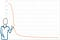Figure 14. Errors per iteration. A total of 10,000 iterations are run. Error drop from 0.4242 to 0.0116

Not bad for ~100 lines of code (you can download the complete source code from my GitHub repository). However, we could have done the same with ~10 lines of code using a library. One of such libraries is Eclipse Deeplearning4j, an open-source, distributed deep-learning library written for Java. We can use a library and solve more complex problems, such as train a neural network for image classification. Inputs will increase, the training data set will be much bigger (than our 4 lines for XOR), and we would need more than one hidden layer. But that is another story. Thanks for reading. Feel free to leave your feedback and reviews below.

# References

Do you want to learn more about details? review here the derivative of the sigmoid function; review here the chain rule in calculus; review here the gradient descendant definition; and here a detailed description of the maths behind backward propagation.

## Analytics Vidhya

Analytics Vidhya is a community of Analytics and Data…

### By Analytics Vidhya

Latest news from Analytics Vidhya on our Hackathons and some of our best articles! Take a look.

Medium sent you an email at to complete your subscription.

Written by

## Javier Gonzalez

Software Engineer, Computer Science Educator, Faculty at @ASUEngineering, and ACM distinguished speaker

## Analytics Vidhya

Analytics Vidhya is a community of Analytics and Data Science professionals. We are building the next-gen data science ecosystem https://www.analyticsvidhya.com

Written by

## Javier Gonzalez

Software Engineer, Computer Science Educator, Faculty at @ASUEngineering, and ACM distinguished speaker## Analytics Vidhya

Analytics Vidhya is a community of Analytics and Data Science professionals. We are building the next-gen data science ecosystem https://www.analyticsvidhya.com

## More From Medium

Medium is an open platform where 170 million readers come to find insightful and dynamic thinking. Here, expert and undiscovered voices alike dive into the heart of any topic and bring new ideas to the surface. Learn more

Follow the writers, publications, and topics that matter to you, and you’ll see them on your homepage and in your inbox. Explore

If you have a story to tell, knowledge to share, or a perspective to offer — welcome home. It’s easy and free to post your thinking on any topic. Write on Medium